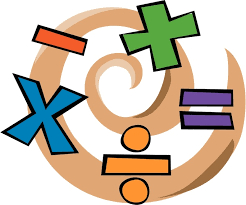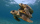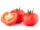# Multiplication negatives

Josh says that (4)(-7)(-1) is equal to (4)(7). Evangelina says (4)(-7)(-1) is equal to (-28)(-1) . Who is correct? Explain your reasoning.

Result

x = (Correct answer is: both)### Step-by-step explanation:Did you find an error or inaccuracy? Feel free to write us. Thank you!## Related math problems and questions:

• Evaluate expression 2Evaluate expression with negatives: (-3)+4+(-8)+(-6)+4+(-1)
• Simplest form of a fractionWhich one of the following fraction after reducing in simplest form is not equal to 3/2? a) 15/20 b) 12/8 c) 27/18 d) 6/4
• Four multiplesFour multiples of 6 he writes the following 12  24  56  72, which is correct?
• Math testBrayden was solving some math problems for the math team. He answered 2 math problems. Matthew answered 3, John answered 1 reasoning. Matthew 1/2 times as many. Brayden said that 2/6. Is he correct? Why or why not? Be sure to explain your answer.
• Equivalent expressionPablo rewrites the expression 8y-24 as 8(y-3). Hana rewrites 8y-24 as 2(4y-12). Are Pablo’s and Hana’s expressions each equivalent to 8y-24? Explain your reasoning.
• Golf ballsOf the 28 golf balls, 1/7 are yellow. How many golf balls are yellow? Use the model to help you. Enter your answer in the box.
• Chocolate chipOut of 28 cookies, 3/4 are chocolate chip. How many cookies are chocolate chip?
• Divide 11Divide the product of 4 and 5/8 by 1 1/2. Write your answer as a mixed number.
• Unknown number 6Determine x if 1/6 of x is equal to 2/5 of the number 24.
• Fractions and mixed numerals(a) Convert the following mixed numbers to improper fractions. i. 3 5/8 ii. 7 7/6 (b) Convert the following improper fraction to mixed number. i. 13/4 ii. 78/5 (c) Simplify these fractions to their lowest terms. i. 36/42 ii. 27/45 2. evaluate following ex
• Three digits number 2Find the number of all three-digit positive integers that can be put together from digits 1,2,3,4 and which are subject to the same time has the following conditions: on one positions is one of the numbers 1,3,4, on the place of hundreds 4 or 2.
• Find the 24Find the difference between 2/7 and 1/21
• Find the 28Find the area of a square with side length 4/5 yard.
• How manyHow many integers are greater than 547/3 and less than 931/4?
• SandySandy, John and Marg baked pies for the Bake Sale. Sandy cut his pies into 6ths, John but his into 8ths and Marg cut hers into quarters. Sandy sold 11/6, John sold  1 3/8 pies and Marg sold 9/4 pies. Who sold the most pies? Who sold the fewest?
• A turtleA turtle is 20 5/6 inches below the surface of a pond. It dives to a depth of 32 1/4 inches. What is the change in the turtle’s position? Write your answer as a mixed number.
• The ketchupIf 3 1/4 of tomatoes are needed to make 1 bottle of ketchup. Find the number of tomatoes required to make 4 1/5 bottles